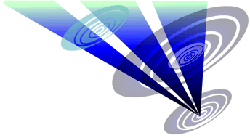Reconstruction of the 2015 Pontecurone, Italy formation 1.Draw a circle. Draw and extend the horizontal and vertical centerlines. 2.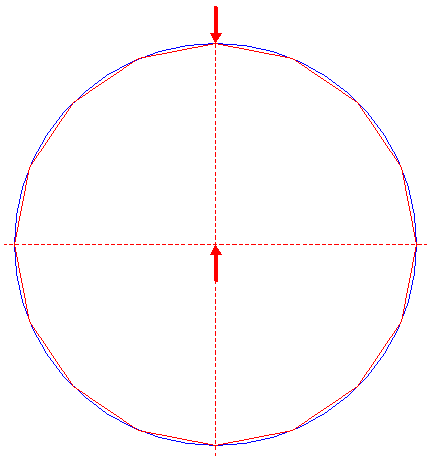Construct the inscribed regular 16-sided polygon of circle 1, pointing up. 3.Draw the sixteen rays of polygon 2, from the center of circle 1 to the angular points. Number these rays clockwise 1 - 16, starting from the one next to the top to the right. 4.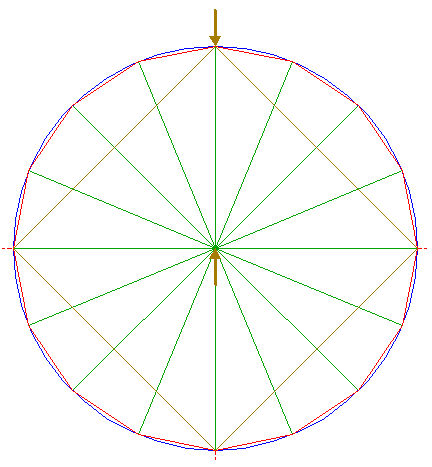Construct the inscribed square of circle 1, pointing up. 5.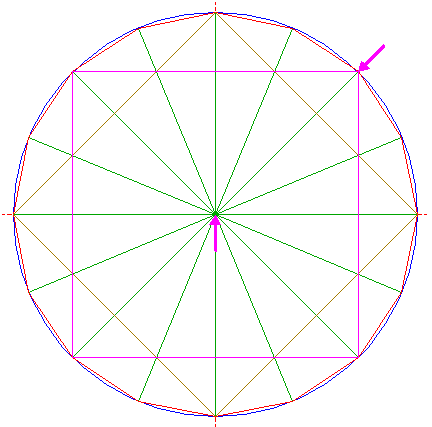Construct the inscribed square of circle 1, with horizontal and vertical sides. 6.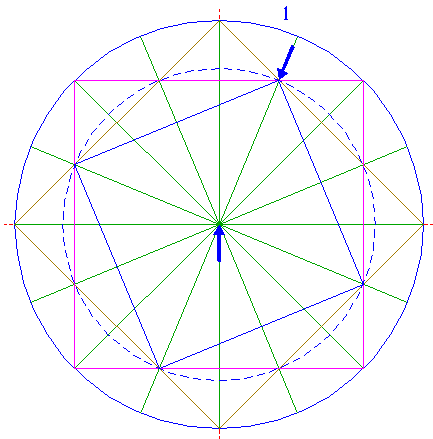Construct a square concentric to circle 1, with one angular point at the intersection of ray 3 nr. 1 and square 5. 7.Construct a square concentric to circle 1, with one angular point at the intersection of ray 3 nr. 15 and square 5. 8.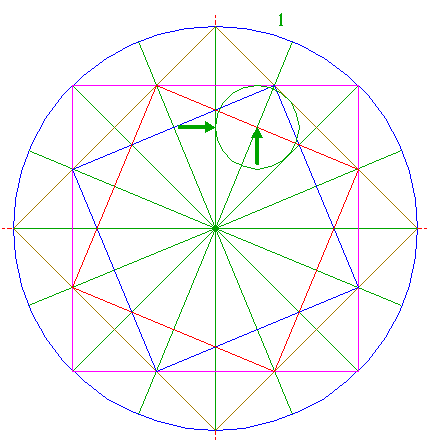Construct a circle centered at the intersection of ray 3 nr. 1 and square 7, tangent to the vertical centerline. 9.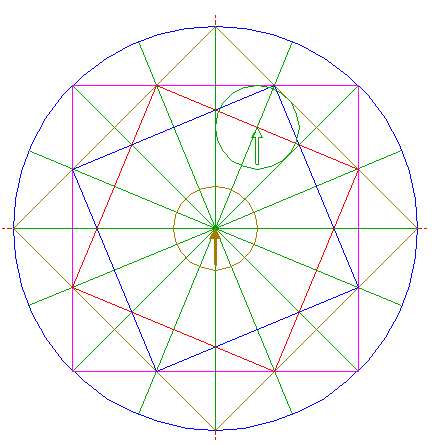Copy circle 8 to the center of circle 1. 10.Construct a circle centered at the intersection of ray 3 nr. 1 and square 5, passing through the intersection (tangent point) of circle 8 and square 5. 11.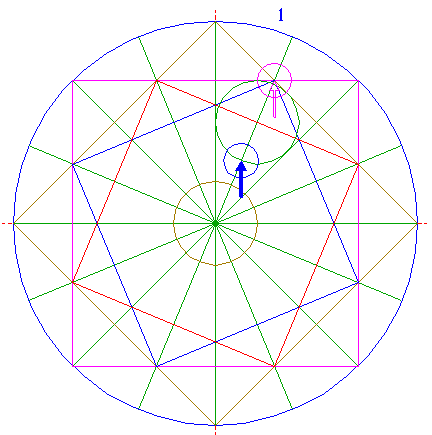Copy circle 10 to the lower intersection of ray 3 nr. 1 and circle 8. 12.Construct a circle concentric to circle 1, tangent to circle 11 at the lower side. 13.Construct a "two-points circle" (defined by the two end-points of a centerline) between the center of circle 9 and its righthand intersection with the horizontal centerline. 14.Copy circle 13 to the center of circle 1. 15.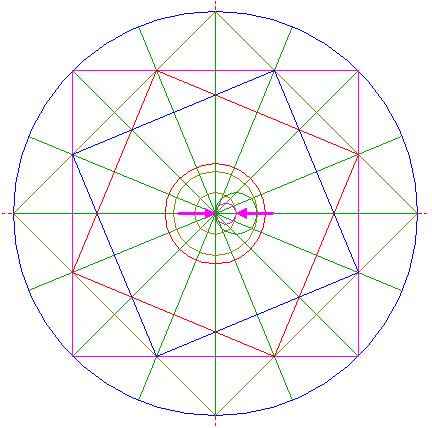Construct a "two-points circle" between the center of circle 14 and its righthand intersection with the horizontal centerline. 16.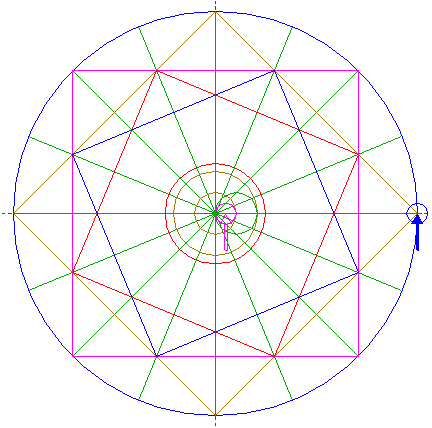Copy circle 15 to the righthand intersection of circle 1 and the horizontal centerline. 17.Construct a circle concentric to circle 1, tangent to circle 16 at the righthand side. 18.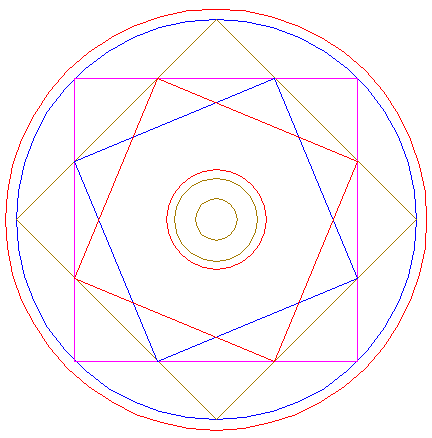Circles 1, 9, 12, 14, and 17, and squares 4, 5, 6, and 7, together make up the final reconstruction. 19.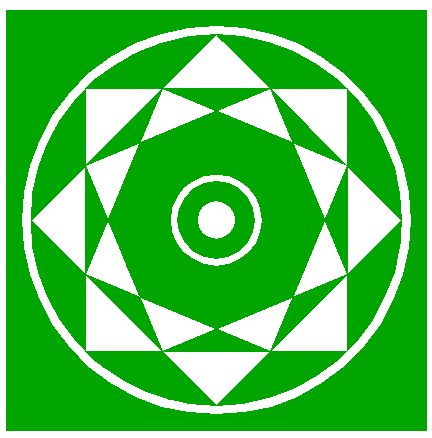Colour all areas corresponding to standing... 20.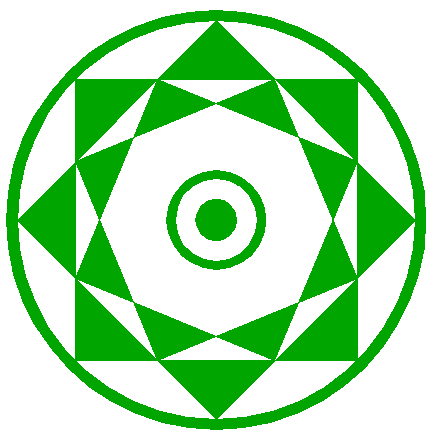...or to flattened crop, and finish the reconstruction of the 2015 Pontecurone, Italy formation. 21.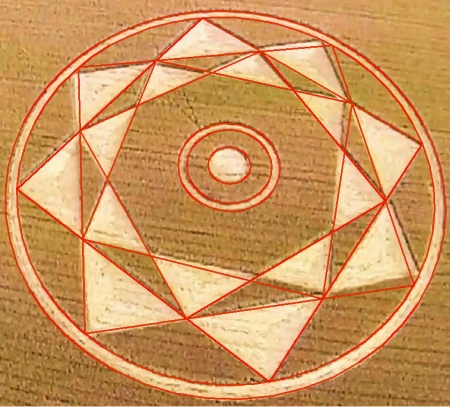The final result, matched with the aerial image.